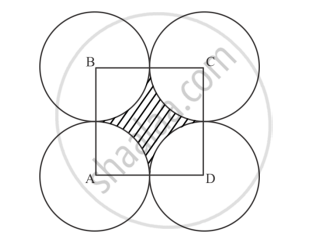Share

# In Below Fig., Points A, B, C and D Are the Centers of Four Circles that Each Have a Radius of Length One Unit. If a Point is Selected at Random from the Interior of Square Abcd. What is the Probability that the Point Will Be Chosen from the Shaded Region? - CBSE Class 10 - Mathematics

ConceptProbability Examples and Solutions

#### Question

In below Fig., points A, B, C and D are the centers of four circles that each have a radius of length one unit. If a point is selected at random from the interior of square ABCD. What is the probability that the point will be chosen from the shaded region?#### Solution

Length of side of square = 1 + 1 = 2cm

Area of square = 2 × 2 = 4cm^2

Area of shaded region = area of square – 4 × area of quadrant

= 4 – 4 (1/4) pi(1)^2

= (4 − 𝜋) cm^2

Probability that the point will be chosen from the shaded region ="Area of shaded regfion"/"Area of square ABCD"= (4−pi)/4 = 1 −(pi/4)

Since geometrical probability,

P(E) ="Measure of specified part of region"/"Measure of the whole region"

Is there an error in this question or solution?

#### Video TutorialsVIEW ALL 

Solution In Below Fig., Points A, B, C and D Are the Centers of Four Circles that Each Have a Radius of Length One Unit. If a Point is Selected at Random from the Interior of Square Abcd. What is the Probability that the Point Will Be Chosen from the Shaded Region? Concept: Probability Examples and Solutions.
S